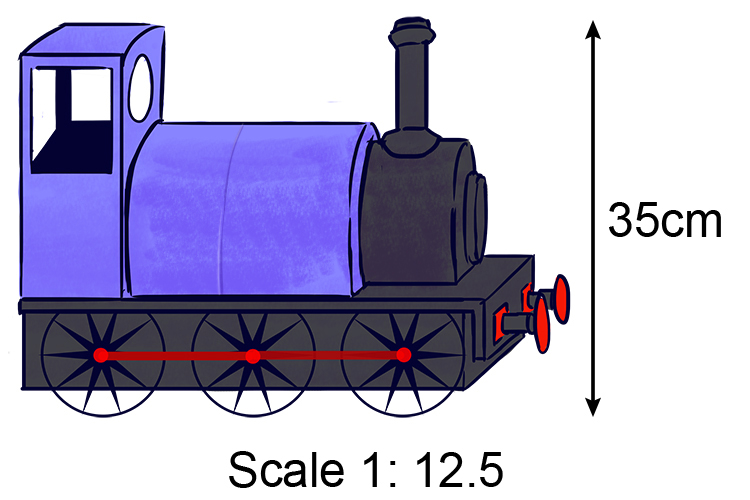# Two part ratios

When you hear the word ratio you must try to remember the words "to every"

If something is 1 to 5 ratio you must read this as 1 to every 5

A ratio shows how two numbers compare.

x to every yRaise a toe (ratio) to every (to every) one.

Ratio = to every

There are several ways mathematicians express ratios

5:1 ratio =5 to every 1

5/1 ratio =5 to every 1

5 to 1 ratio =5 to every 1

Example 1

A train has been built at a ratio of 1:12.5. If the model train is 35cm tall how tall is the real thing?The ratio is 1 to every 12.5

so 1cm to every 12.5cm

If         1=12.5

then 35=x

(treat this like all calculations in our percentages section)

Put a divide sign between them

1/35=12.5/x

rearranging

x=(12.5times35)/1

x=437.5cm

The real train is 437.5cm tall.

Example 2

To make biscuits you need 350\ grams of wheat. This makes 22 biscuits. How many grams of wheat do you need to make 30 biscuits?

So the ratio is 22 biscuits to every 350\ grams wheat.

22=350

Work out 1 biscuit

1=x

(treat this like all the calculations in our percentages section).

Put a divide sign between them

22/1=350/x

rearrange

x=(350times1)/22

x=15.9\ grams of wheat.

so the ratio is

1 biscuit to every 15.9\ grams of wheat

Now we can work out 30 biscuits.

1=15.9

30=x

(treat this like all the calculations in our percentages section).

Put a divide sign between them.

1/30=15.9/x

rearranging

x=(15.9times30)/1

x=477\ grams

477\ grams make 30 biscuits.